Like   Pin   +1   in# Let's look around my studio together.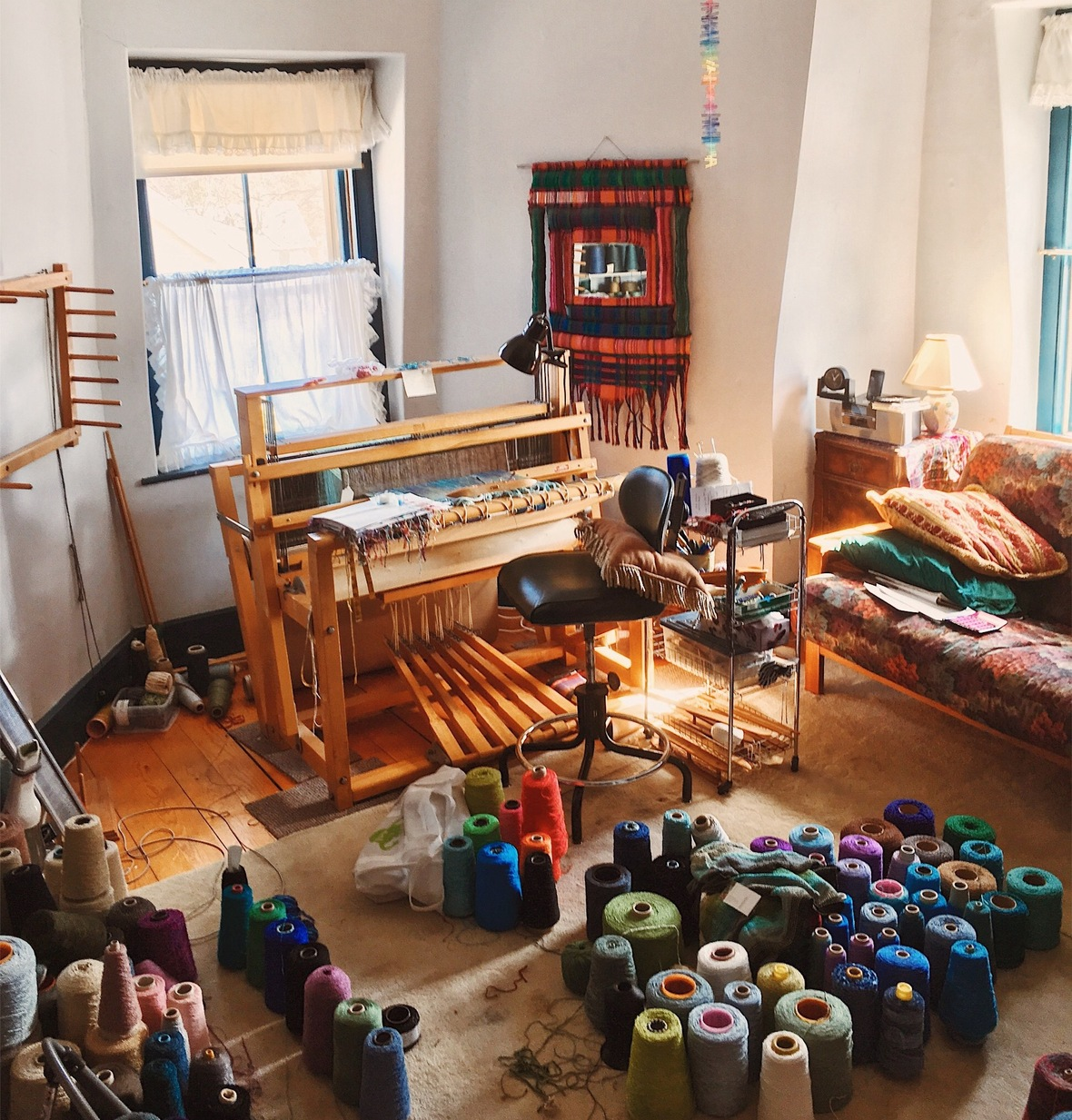The space seems random but is actually quite organized for me: yarns on the floor, my couch for great afternoon naps in the sun, loom and warping board set up to go. This space isn't just filled with my own work materials, it also holds pieces that inspire me and keep me motivated. These are things that make me happy!

## Here are a few of my favorite objects.

 table div table+table+table+table+table div table{width:100%;padding:0}table div table+table+table+table+table div table img{width:96.23%;padding:0;float:none}table div table+table+table+table+table div table td{width:100%;padding:0 1.88% 18px}/* styles */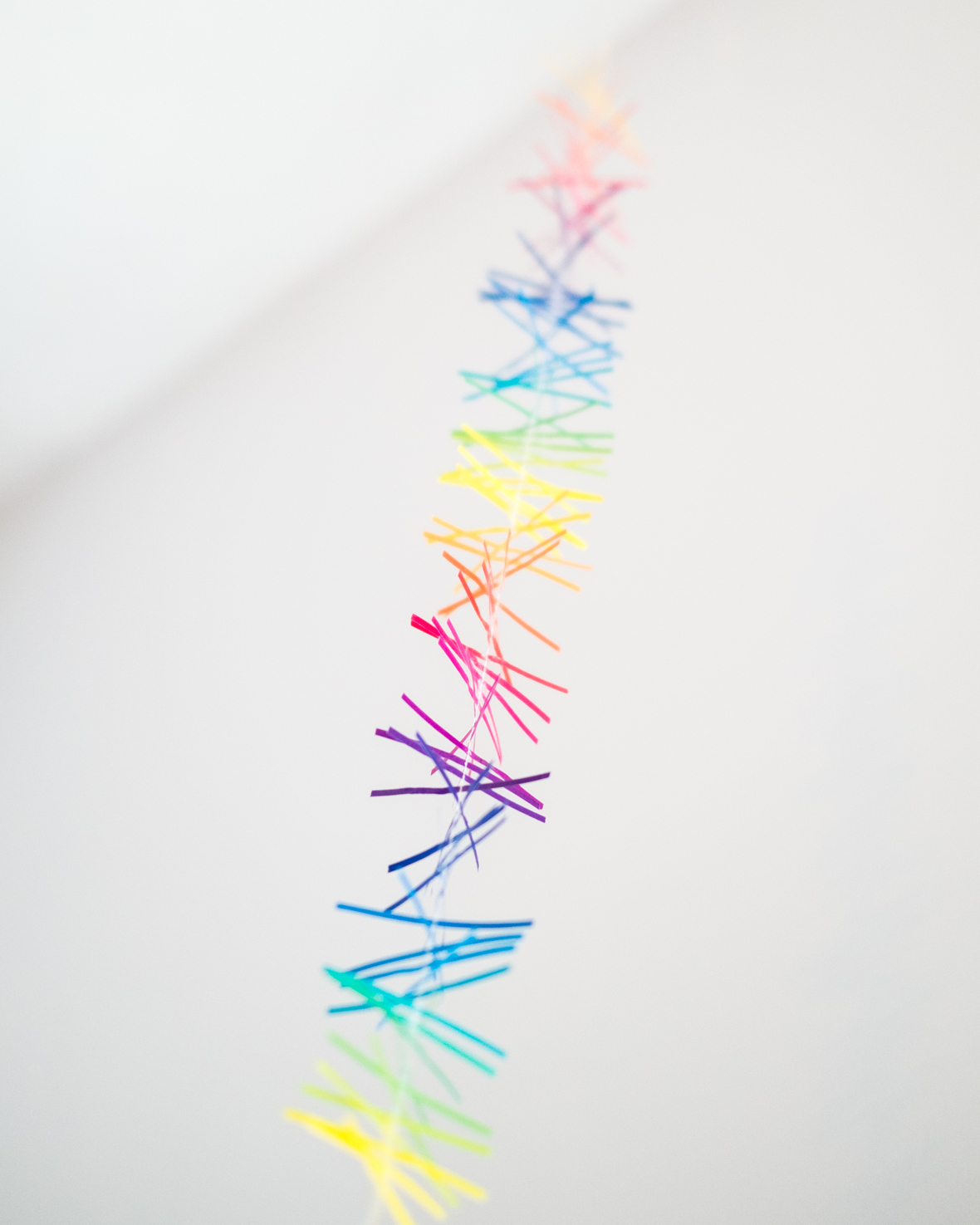Love how this flutters in the breeze.

This is a rainbow mobile from the deCordova Museum shop. I often end up purchasing pieces when I drop off my work at galleries and shops. Now I have an interesting collection of pieces from all over the place.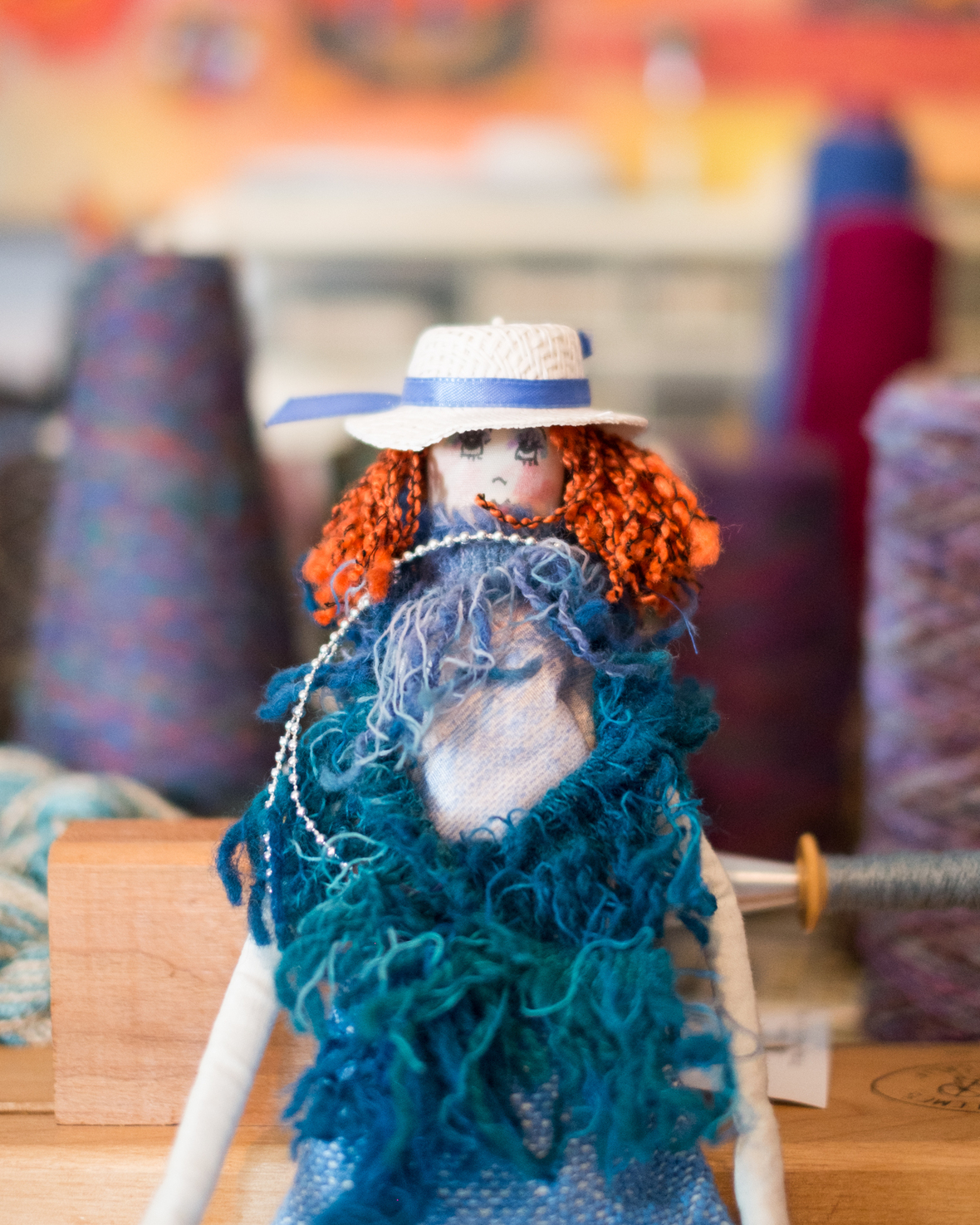Handmade doll by Lois Hardy, Weaver's Guild of Boston member.

Here's a great find from the Weaver's Guild of Boston annual sale. The dolls were fanciful and full of personality. This one is a "shopper!"

 table div table+table+table+table+table+table+table+table div table{width:100%;padding:0}table div table+table+table+table+table+table+table+table div table img{width:96.23%;padding:0;float:none}table div table+table+table+table+table+table+table+table div table td{width:100%;padding:0 1.88% 18px}/* styles */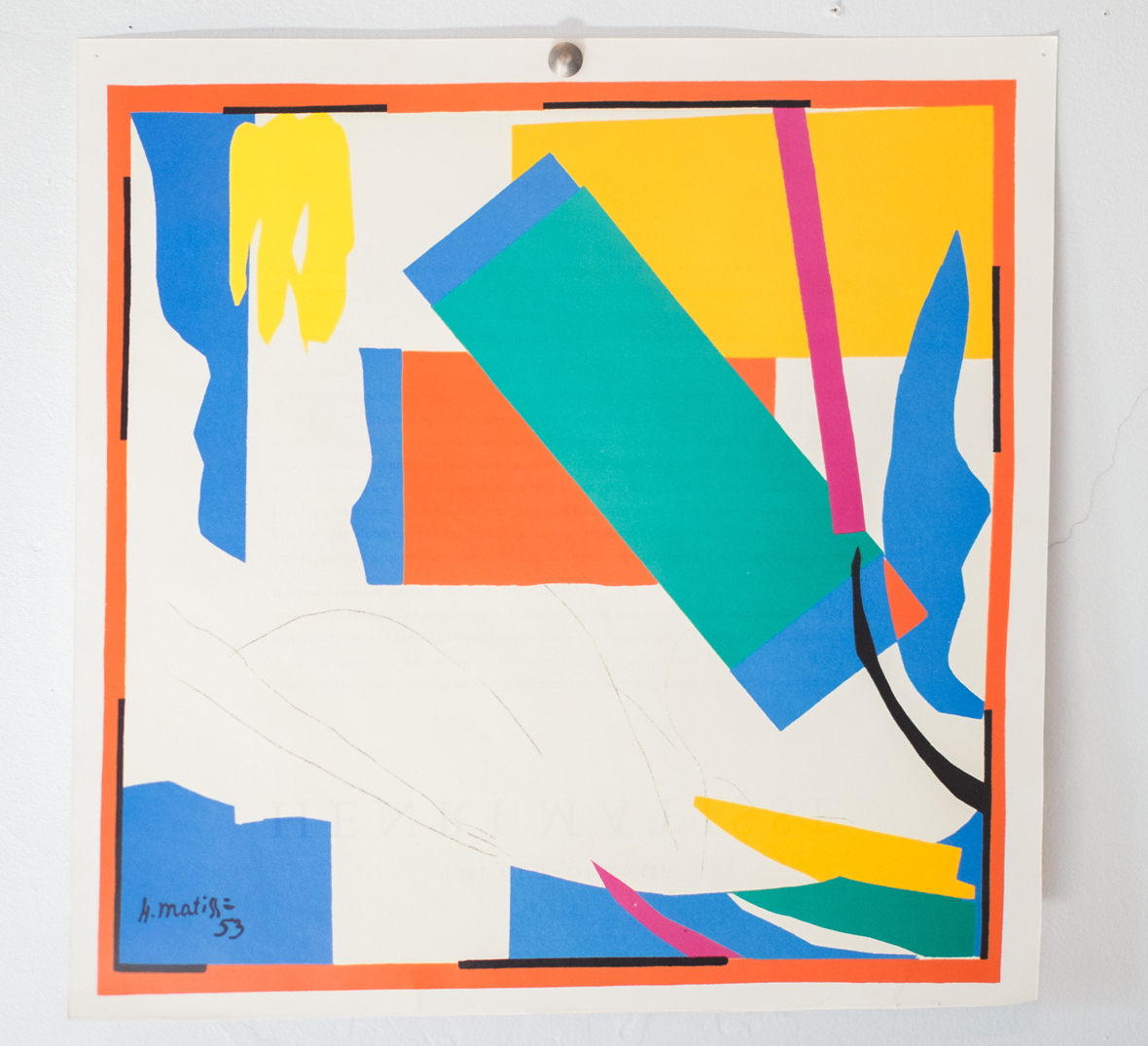Matisse is one of my all time favorite artists. Calendar page from a prior year.
 table div table+table+table+table+table+table+table+table+table+table div table{width:100%;padding:0}table div table+table+table+table+table+table+table+table+table+table div table img{width:96.23%;padding:0;float:none}table div table+table+table+table+table+table+table+table+table+table div table td{width:100%;padding:0 1.88% 18px}/* styles */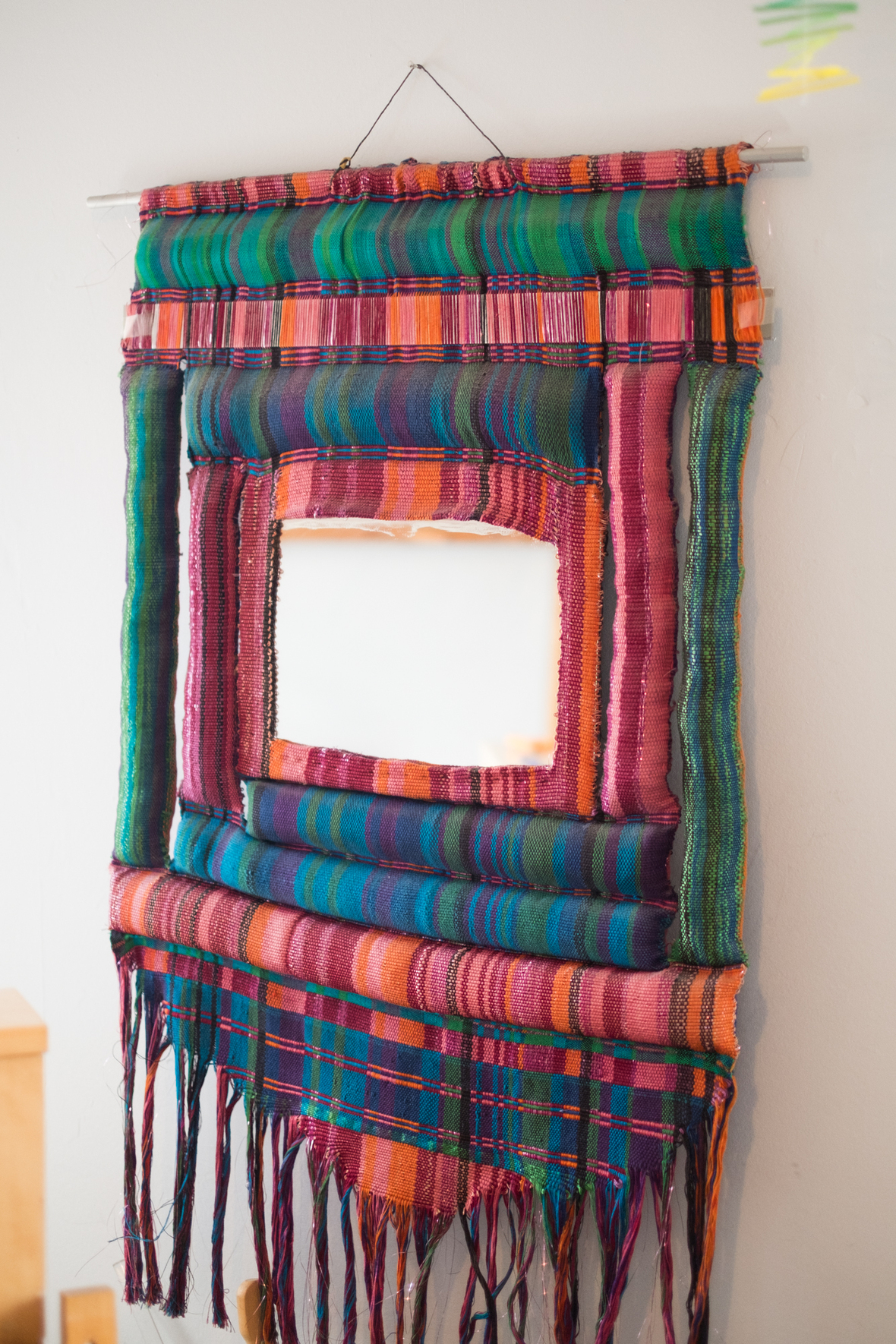Handwoven, Hetty Friedman 1975

This piece hung in the front hall of my parent's Brooklyn home from 1975-1998. It's the first piece I wove when I showed up at SMU (now UMass Dartmouth) for my MFA. I had woven this with cotton yarns I had brought back from Guatemala.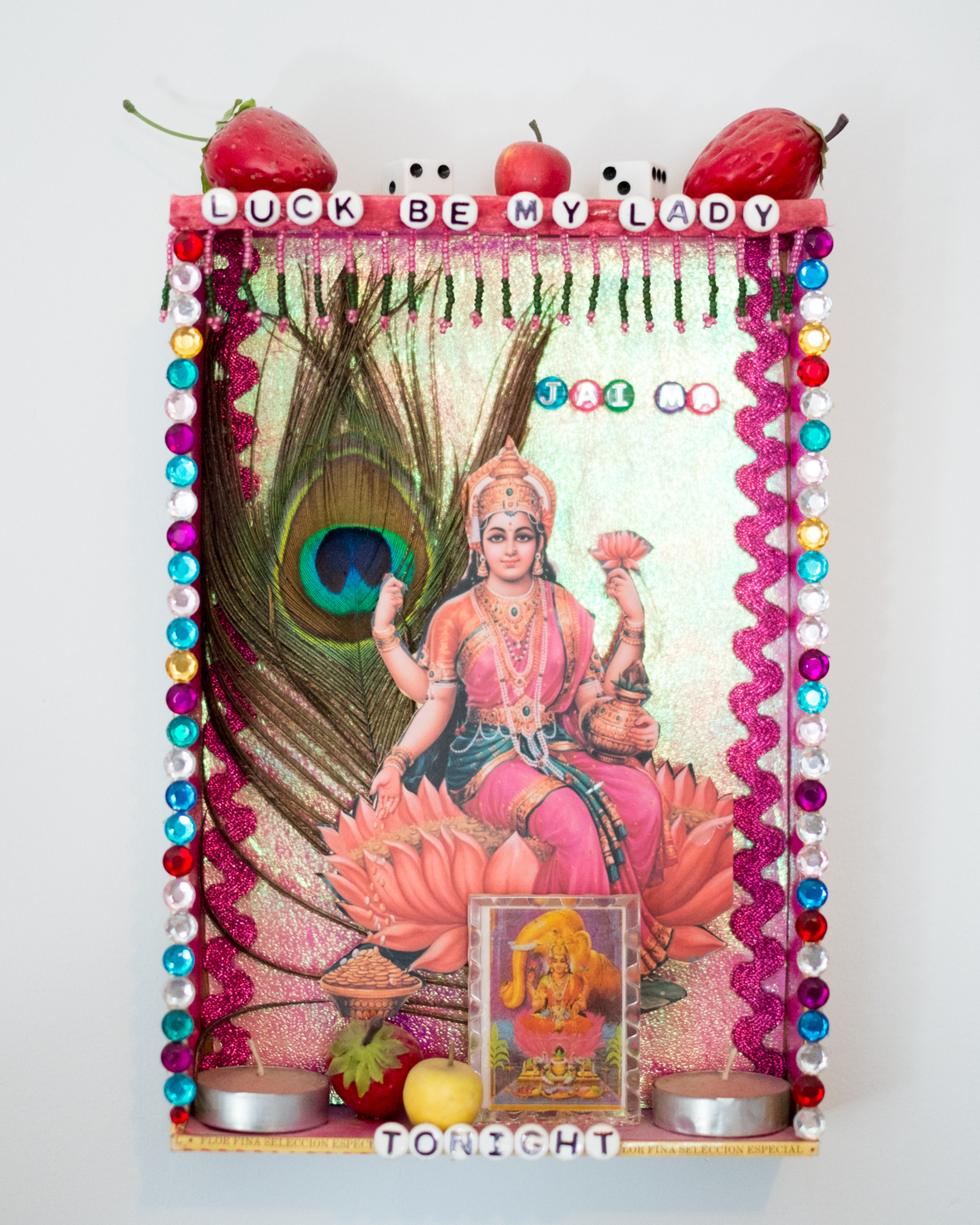Annapurna, Taos 2005. Artist unknown

I purchased this shadow box piece in Taos, in 2006 (I was on a retreat studying with Natalie Goldberg at the time). I loved the words, the colors and the overall sentiment–textured, fun and quirky. See the tea lamps?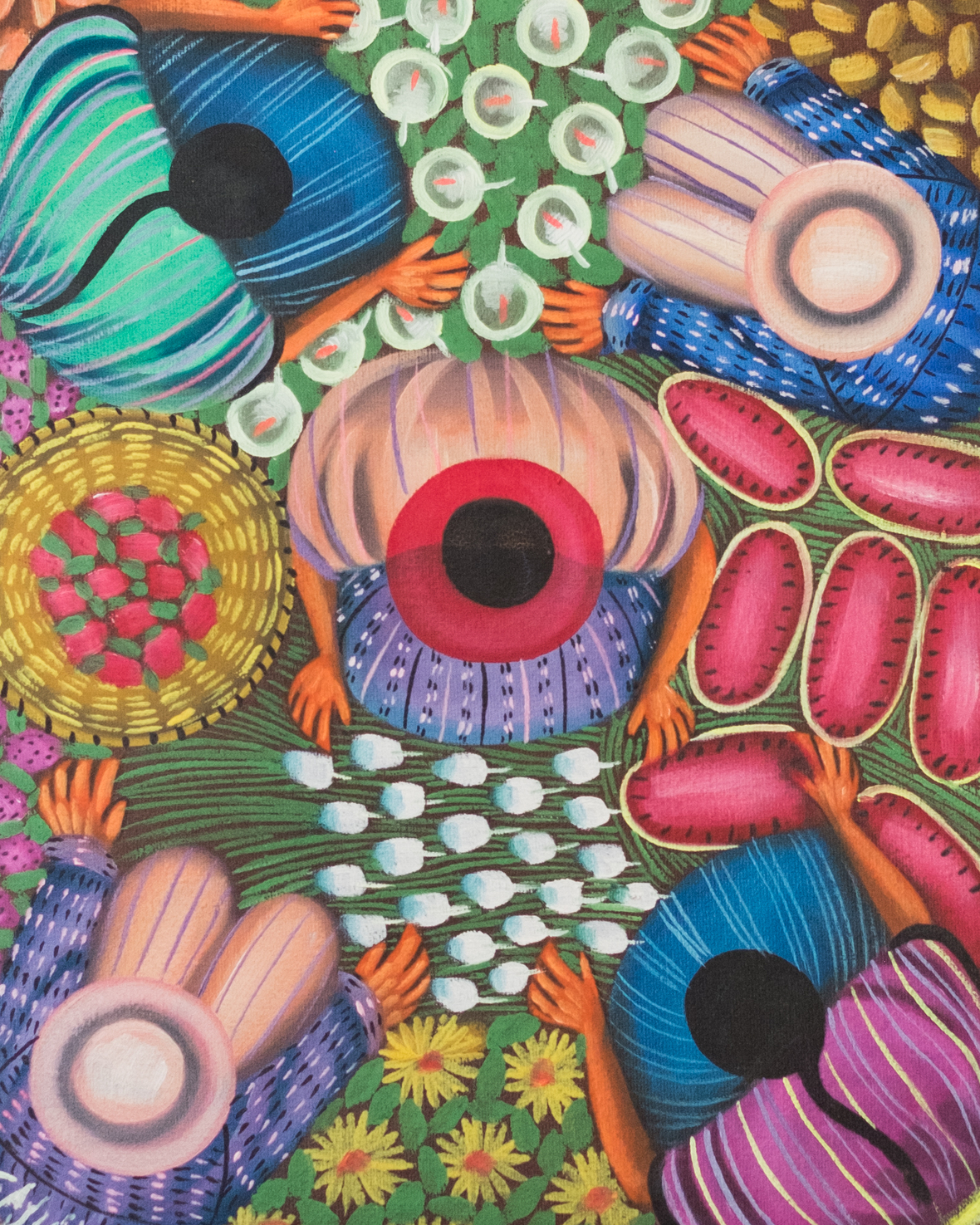Angelina Quic Ixtamer, San Juan Atitlan, Guatemala

One of Angelina Quic Ixtamer's fabulous paintings. I collect these each time I am on tour in Guatemala. She invented this bird's eye view of painting. Her colors, textures, and themes resonate for me.

 table div table+table+table+table+table+table+table+table+table+table+table+table+table+table div table{width:100%;padding:0}table div table+table+table+table+table+table+table+table+table+table+table+table+table+table div table img{width:96.23%;padding:0;float:none}table div table+table+table+table+table+table+table+table+table+table+table+table+table+table div table td{width:100%;padding:0 1.88% 18px}/* styles */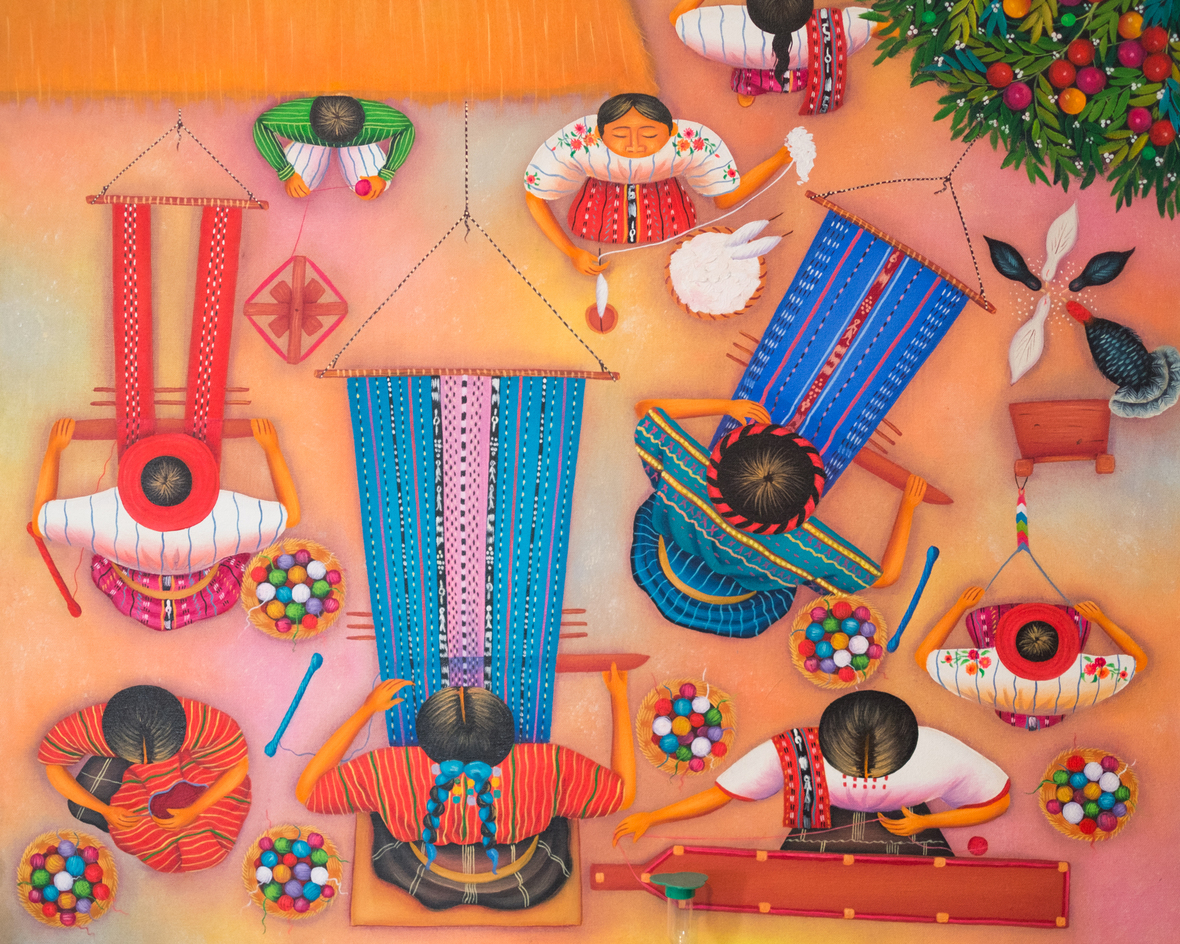Angelina Quic Ixtamer, San Juan Atitlan, Guatemala

## What do you hold dear? What fills your creative spaces?

Let me know your thoughts. I'd love to hear from you.

 table div table+table+table+table+table+table+table+table+table+table+table+table+table+table+table+table+table div table{width:100%;padding:0}table div table+table+table+table+table+table+table+table+table+table+table+table+table+table+table+table+table div table img{width:96.23%;padding:0;float:none}table div table+table+table+table+table+table+table+table+table+table+table+table+table+table+table+table+table div table td{width:100%;padding:0 1.88% 18px}/* styles */# Looking for a present that will catch her eye?

It's not too late: use offer code MOM15 to get 15% off, now until May 12. Don't forget to check my sale section, where I've collected lots of old favorites. Browse through bamboo and rayon woven pieces, plus unique jewelry your mother–or you!–will enjoy.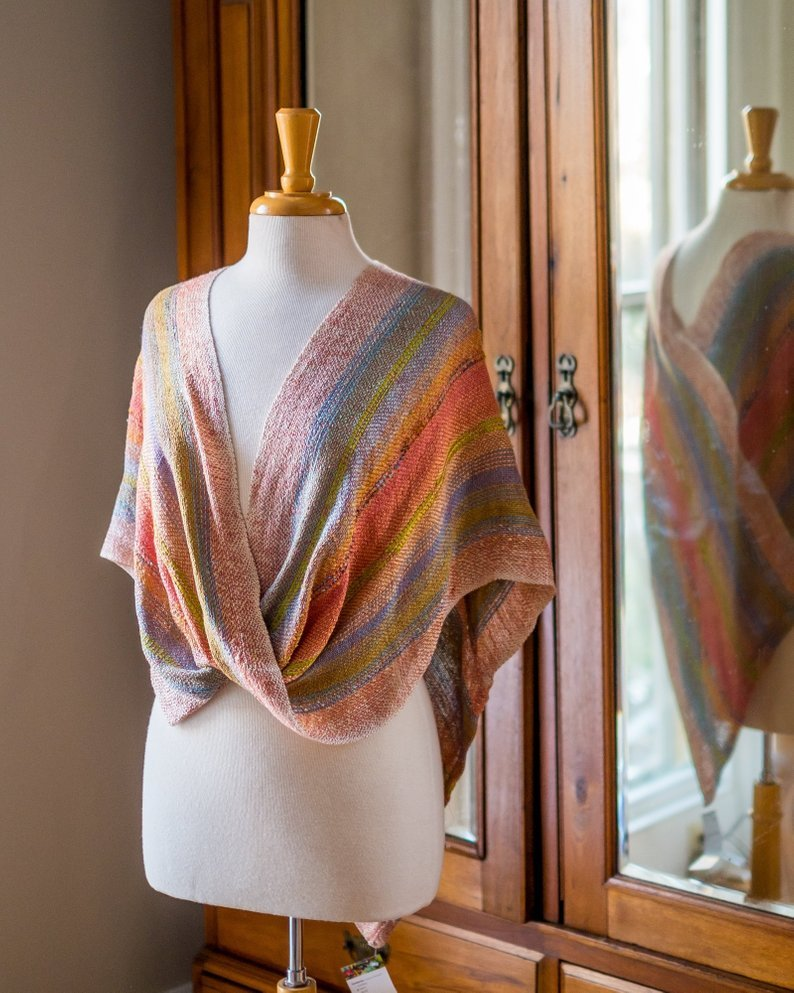Coral, lime, and blue mobius shawl with cream borders.
 table div table+table+table+table+table+table+table+table+table+table+table+table+table+table+table+table+table+table+table+table+table div table{width:100%;padding:0}table div table+table+table+table+table+table+table+table+table+table+table+table+table+table+table+table+table+table+table+table+table div table img{width:96.23%;padding:0;float:none}table div table+table+table+table+table+table+table+table+table+table+table+table+table+table+table+table+table+table+table+table+table div table td{width:100%;padding:0 1.88% 18px}/* styles */# Come say hello!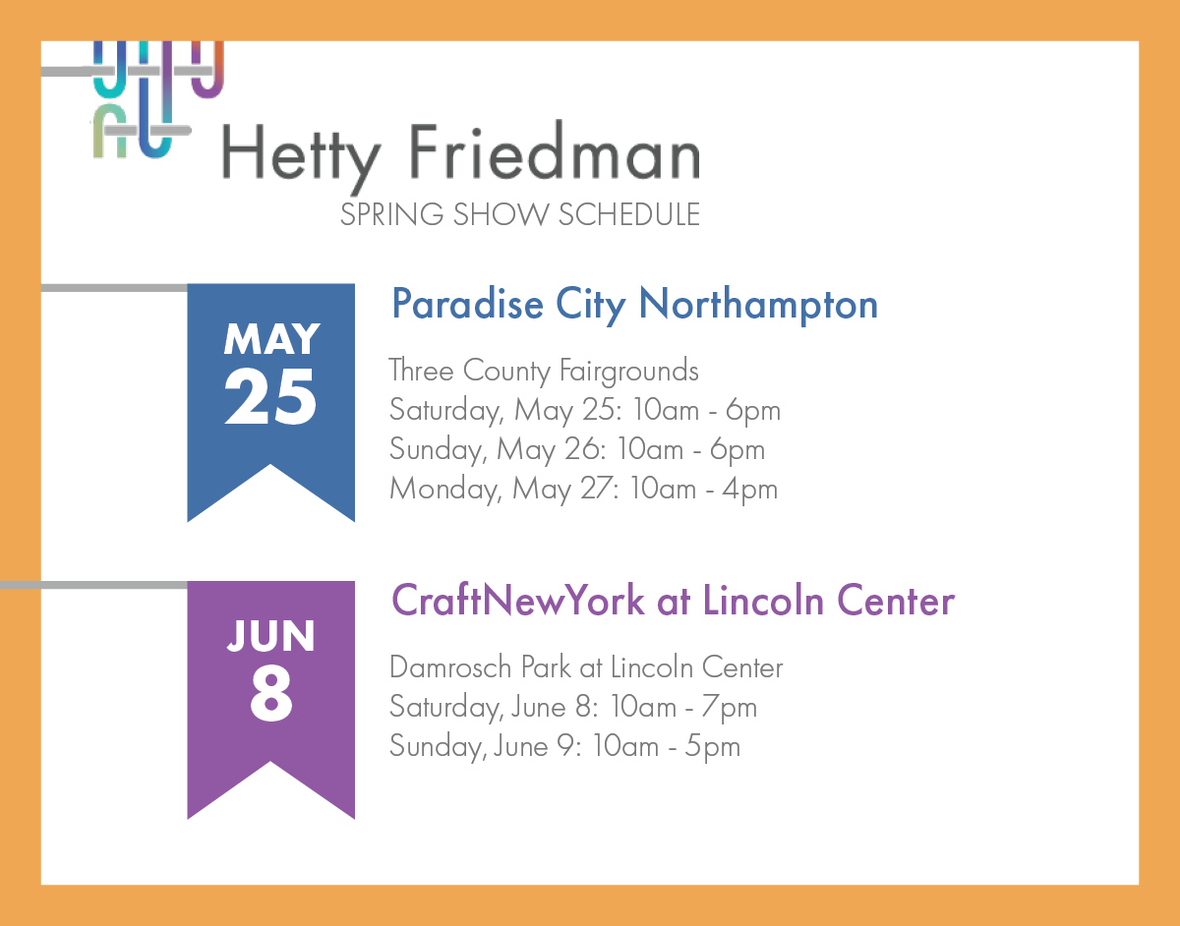table div table+table+table+table+table+table+table+table+table+table+table+table+table+table+table+table+table+table+table+table+table+table+table+table div table{width:100%;padding:0}table div table+table+table+table+table+table+table+table+table+table+table+table+table+table+table+table+table+table+table+table+table+table+table+table div table img{width:96.23%;padding:0;float:none}table div table+table+table+table+table+table+table+table+table+table+table+table+table+table+table+table+table+table+table+table+table+table+table+table div table td{width:100%;padding:0 1.88% 18px}/* styles */# Don't miss a thing.

Click the icons down below to like, follow, and share all my social media accounts. I'm on Facebook, Instagram, and Pinterest with behind-the-scenes photos, thought provoking articles, and special offers.
Get there by clicking the icons down below. And thank you for helping me out by sharing my newsletter!

 table div table+table+table+table+table+table+table+table+table+table+table+table+table+table+table+table+table+table+table+table+table+table+table+table+table+table div table{width:100%;padding:0}table div table+table+table+table+table+table+table+table+table+table+table+table+table+table+table+table+table+table+table+table+table+table+table+table+table+table div table table{padding:0;float:left!important;width:34.622%!important}table div table+table+table+table+table+table+table+table+table+table+table+table+table+table+table+table+table+table+table+table+table+table+table+table+table+table div table td{padding-left:6px;padding-right:6px}table div table+table+table+table+table+table+table+table+table+table+table+table+table+table+table+table+table+table+table+table+table+table+table+table+table+table div table table+table{float:left!important;width:34.622%!important}table div table+table+table+table+table+table+table+table+table+table+table+table+table+table+table+table+table+table+table+table+table+table+table+table+table+table div table table td,table div table+table+table+table+table+table+table+table+table+table+table+table+table+table+table+table+table+table+table+table+table+table+table+table+table+table div table table+table td{padding-left:0;padding-right:20px}table div table+table+table+table+table+table+table+table+table+table+table+table+table+table+table+table+table+table+table+table+table+table+table+table+table+table div table table+table+table{float:left!important;width:30.756%!important}table div table+table+table+table+table+table+table+table+table+table+table+table+table+table+table+table+table+table+table+table+table+table+table+table+table+table div table table+table+table td{padding-left:0;padding-right:0}/* styles */
 table div table+table+table+table+table+table+table+table+table+table+table+table+table+table+table+table+table+table+table+table+table+table+table+table+table+table+table div table{width:100%;padding:0}table div table+table+table+table+table+table+table+table+table+table+table+table+table+table+table+table+table+table+table+table+table+table+table+table+table+table+table div table img{width:96.23%;padding:0;float:none}table div table+table+table+table+table+table+table+table+table+table+table+table+table+table+table+table+table+table+table+table+table+table+table+table+table+table+table div table td{width:100%;padding:0 1.88% 18px}/* styles */# Have you forgotten all the ways to wear your mobius shawl?

## Check out this video and pass it along to your friends!

 table div table+table+table+table+table+table+table+table+table+table+table+table+table+table+table+table+table+table+table+table+table+table+table+table+table+table+table+table+table div table{width:100%;padding:0}table div table+table+table+table+table+table+table+table+table+table+table+table+table+table+table+table+table+table+table+table+table+table+table+table+table+table+table+table+table div table img{width:96.23%;padding:0;float:none}table div table+table+table+table+table+table+table+table+table+table+table+table+table+table+table+table+table+table+table+table+table+table+table+table+table+table+table+table+table div table td{width:100%;padding:0 1.88% 18px}/* styles */## Visit my website: hettyfriedmandesigns.com

Email: hetty.friedman@gmail.com Phone: 617.512.5344